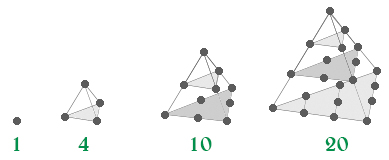# Tetrahedral Numbers

A number is termed as a tetrahedral number if it can be represented as a pyramid with a triangular base and three sides, called a tetrahedron. The nth tetrahedral number is the sum of the first n triangular numbers.

The first ten tetrahedral numbers are:
1, 4, 10, 20, 35, 56, 84, 120, 165, 220, …## Recommended: Please try your approach on {IDE} first, before moving on to the solution.

Formula for nth tetrahedral number:

```Tn = (n * (n + 1) * (n + 2)) / 6
```

Proof:

```The proof uses the fact that the nth tetrahedral
number is given by,

Trin = (n * (n + 1)) / 2

It proceeds by induction.

Base Case
T1 = 1 = 1 * 2 * 3 / 6

Inductive Step
Tn+1 = Tn + Trin+1

Tn+1 = [((n * (n + 1) * (n + 2)) / 6] + [((n + 1) * (n + 2)) / 2]

Tn+1 = (n * (n + 1) * (n + 2)) / 6
```

Below is the implementation of above idea :

## C++

 `// CPP Program to find the ` `// nth tetrahedral number ` `#include ` `using` `namespace` `std; ` ` `  `int` `tetrahedralNumber(``int` `n) ` `{ ` `    ``return` `(n * (n + 1) * (n + 2)) / 6; ` `} ` ` `  `// Driver Code ` `int` `main() ` `{ ` `    ``int` `n = 5; ` `     `  `    ``cout << tetrahedralNumber(n) << endl; ` ` `  `    ``return` `0; ` `} `

## Java

 `// Java Program to find the ` `// nth tetrahedral number ` `class` `GFG { ` `     `  `// Function to find Tetrahedral Number ` `static` `int` `tetrahedralNumber(``int` `n) ` `{ ` `    ``return` `(n * (n + ``1``) * (n + ``2``)) / ``6``; ` `} ` ` `  `// Driver Code ` `public` `static` `void` `main(String[] args) ` `{ ` `    ``int` `n = ``5``; ` `     `  `    ``System.out.println(tetrahedralNumber(n)); ` `} ` `} ` ` `  `// This code is contributed by Manish Kumar Rai. `

## Python

 `# Python3 Program to find the ` `# nth tetrahedral number ` ` `  `def` `tetrahedralNumber(n): ` `     `  `    ``return` `(n ``*` `(n ``+` `1``) ``*` `(n ``+` `2``)) ``/` `6` ` `  `# Driver Code ` `n ``=` `5` `print` `(tetrahedralNumber(n)) `

## C#

 `// C# Program to find the ` `// nth tetrahedral number ` `using` `System; ` ` `  `public` `class` `GFG{ ` `     `  `    ``// Function to find Tetrahedral Number ` `    ``static` `int` `tetrahedralNumber(``int` `n) ` `    ``{ ` `        ``return` `(n * (n + 1) * (n + 2)) / 6; ` `    ``} ` `     `  `    ``// Driver code ` `    ``static` `public` `void` `Main () ` `    ``{ ` `        ``int` `n = 5; ` `     `  `        ``Console.WriteLine(tetrahedralNumber(n)); ` `    ``} ` `} ` ` `  `// This code is contributed by Ajit. `

## PHP

 ` `

Output:

```35
```

Time Complexity: O(1).

Attention reader! Don’t stop learning now. Get hold of all the important DSA concepts with the DSA Self Paced Course at a student-friendly price and become industry ready.

My Personal Notes arrow_drop_upCheck out this Author's contributed articles.

If you like GeeksforGeeks and would like to contribute, you can also write an article using contribute.geeksforgeeks.org or mail your article to contribute@geeksforgeeks.org. See your article appearing on the GeeksforGeeks main page and help other Geeks.

Please Improve this article if you find anything incorrect by clicking on the "Improve Article" button below.

Improved By : Manish_100, jit_t, Mithun Kumar